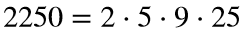# Problem 58019. Factor a number into Fermi-Dirac primes

Cody Problem 58018 asked you to list the Fermi-Dirac primes, which are prime powers with exponents that are powers of 2. As noted there, the name comes from an analogy with particle physics because every number can be written as the product of a unique subset of the Fermi-Dirac primes. For example,.
Write a function analogous to factor(n) that factors the number n into Fermi-Dirac primes. List the Fermi-Dirac primes in ascending order. Take the factorization of 1 to be 1.

### Solution Stats

100.0% Correct | 0.0% Incorrect
Last Solution submitted on Oct 17, 2023

### Community Treasure Hunt

Find the treasures in MATLAB Central and discover how the community can help you!

Start Hunting!